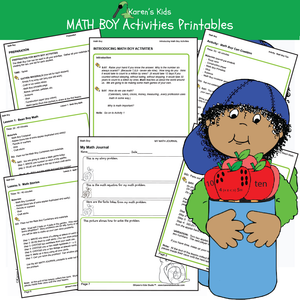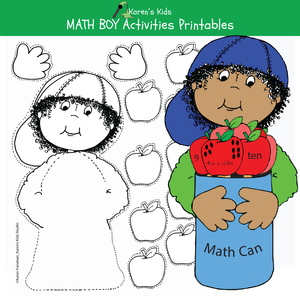Free MATH BOY Activity Kit

Regular price
\$0.00
Sale price
\$0.00

Practice number recognition, counting, matching, sequence, number words, adding, subtracting, multiplying and story problems.  Adjust the lessons for various ages and academic levels from Pre-K to 3rd grade.

Description

Eight pages
Format: PDF

File Type:  Compressed Zip File    File Size:  1 MB

Math Boy Lessons Set
Preparation for Math Activities
Introducing Math Project to Students
Activity:  Math Boy Can Creation
Lesson 1:  Bean Math
Lesson 2:  Memory Math Math
Lesson 3:  Math Stories
My Math Journal
Math Boy_Apple Cut Out Templates